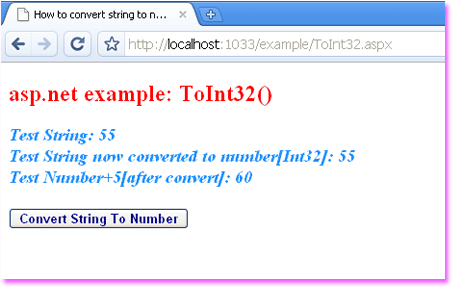### c# - How to convert a string to int

Convert.ToInt32() Method - Convert string to numeric value
.net Convert class allow us to convert a base data type to another base data type. Convert class ToInt32(String) method converts the specified string representation of a number to an equivalent 32 bit signed integer.

Convert.ToInt32(String) overloaded method require to pass a parameter. this parameter name is 'value' which data type is System.String. this parameter pass the string that contains the number to convert. this method return a 32 bit signed integer that is equivalent to the number in value. if the parameter value is null then the method return zero (0).

we also can convert a string object to numeric value (int 32 bit integer) by using Int32.Parse(String) method or Int32.TryParse method. Int32.TryParse method returns an additional value that indicate whether the conversion succeed.

the following asp.net c# example code demonstrate us how can we convert a string to int32 numeric value in .net framework.
ToInt32.aspx
``````
<%@ Page Language="C#" %>

<!DOCTYPE html>

<script runat="server">
protected void Button1_Click(object sender, System.EventArgs e) {
string testString = "55";
int testNumber = Convert.ToInt32(testString);
int sum = testNumber + 5;
Label1.Text = "Test String: " + testString;
Label1.Text += "<br />Test String now converted to number[Int32]: " + testNumber;
Label1.Text += "<br />Test Number+5[after convert]: " + sum;
}
</script>

<html xmlns="http://www.w3.org/1999/xhtml">
<title>How to convert string to numeric value (number ToInt32) in asp.net</title>
<body>
<form id="form1" runat="server">
<div>
<h2 style="color:Red">asp.net example: ToInt32()</h2>
<asp:Label
ID="Label1"
runat="server"
Font-Size="Large"
ForeColor="DodgerBlue"
Font-Bold="true"
Font-Italic="true"
>
</asp:Label>
<br /><br />
<asp:Button
ID="Button1"
runat="server"
OnClick="Button1_Click"
Font-Bold="true"
Text="Convert String To Number"
ForeColor="DarkBlue"
/>
</div>
</form>
</body>
</html>
``````More c# examples